TitleCollege Algebra
Answer/Discussion to Practice Problems
Tutorial
47: Exponential Growth and DecayAnswer/Discussion to 1a The value of the property in a particular block follows a pattern of exponential growth.  In the year 2001, your company purchased a piece of property in this block.  The value of the property in thousands of dollars, t years after 2001 is given by the exponential growth model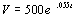A) What did your company pay for the property?

 Since we are looking for the value of the property, what variable are we seeking?  If you said V you are right on!!!! Note that even though we are using V instead of A, the concept of and formula for exponential growth is the same as discussed in the lesson. The way the problem is worded, 2001 is what we call our initial year.  This is where t = 0.  Plugging in 0 for t and solving for V we get: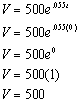*Replace t with 0 *e^0 simplifies to be 1

 While writing up the final answer, keep in mind that the problem said the value was in thousands of dollars.   In 2001, the value of the property was \$500,000. We could have also approached this problem by noting that the year was 2001, which is our initial year, so basically it was asking us for the initial worth, which is Vo (Ao in the formula).  This happens to be the number in front of e which is 500 in this problem.    The reason I showed you using the formula was to get you use to it.  Just note that when it is the initial year, t is 0, so you will have e raised to the 0 power which means it will simplify to be 1 and you are left with whatever Vo (Ao) is.

 B) By what percentage is the price of the property in this block increasing per year?

 In the general growth formula, k is a constant that represents the growth rate. k is the coefficient of t in e’s exponent. So what would be our answer in terms of percent? Well, k = .055, so converting that to percent we get 5.5% for our answer.

 C) What will the property be worth in the year 2010?

 Since we are looking for the population, what variable are we looking for?  If you said V give yourself a high five. What are we going to plug in for t in this problem? Our initial year is 2001, and since t represents years after 2010, we can get t from 2010 - 2001, which would be 9. Plugging in 9 for t and solving for V we get: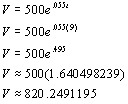*Replace t with 9 *e^ .495 is approx. 1.640498239

 Keep in mind that the problem said that the value was in thousands of dollars.   The value of the property in 2010 would be approximately \$820,249.12.

 D) When will the property be worth 750 thousand dollars?

 Again, it looks like we have a little twist here.  Now we are given the value of the property and we need to first find t to find out how many years after 2001 we are talking about and then convert that knowledge into the actual year. We will still be using the same formula we did to answer the questions above, we will just be using it to find a different variable. Plugging in 750 for V and solving for t we get: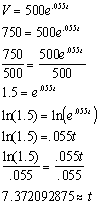*Replace V with 750 *Take the ln of both sides *Use Inv Prop I of Logs to simplify

 This means a little over 7 years after 2001, the value of the property will be 750 thousand dollars. So our answer is during the year 2008.Answer/Discussion to 1b An artifact originally had 10 grams of carbon-14 present.  The decay modeldescribes the amount of carbon-14 present after t years.

 A) How many grams of carbon-14 will be present in this artifact after 25,000 years?

 What are we going to plug in for t in this problem? Since t represents the number of years, it looks like we will be plugging in 25,000 for t. Plugging in 25000 for t and solving for A we get: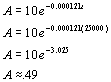*replace t with 25000

 There will be approximately .49 grams of carbon-14 present after 25,000 years.

 B) What is the half-life of carbon-14?

 If we are looking for the half-life of carbon-14, what variable are we looking for?  If you said t give yourself a high five!!!!   It looks like we don’t have any values to plug into A.  However, the problem did say that we were interested in the HALF-life, which would  mean ½ of the original amount (10) would be present at the end (A).  This means A can be replaced with 10/2 = 5. Replacing A with 5 and solving for t we get: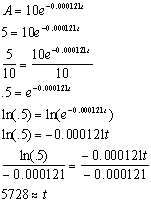*Replace A with 5 *Take the ln of both sides *Use Inv Prop I of Logs to simplify

 The half-life of carbon-14 is approximately 5728 years.

Last revised on March 23, 2011 by Kim Seward.
All contents copyright (C) 2002 - 2011, WTAMU and Kim Seward. All rights reserved.